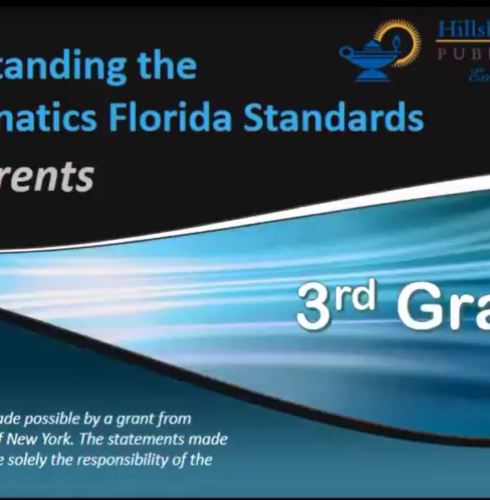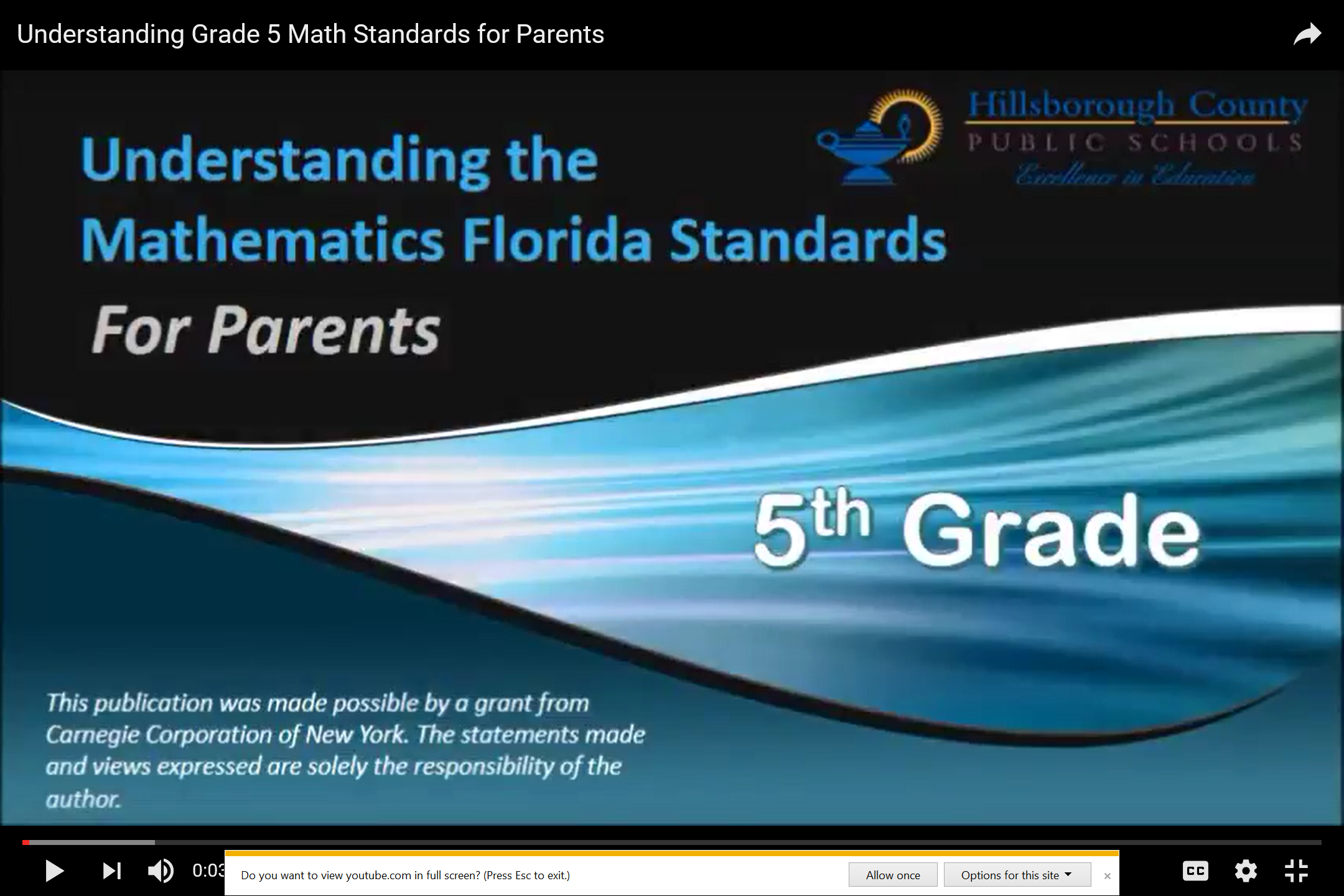What is multiplication?

Multiplication is when we know the number of groups and the size of the equal groups, but the total amount is unknown. Students are expected to use the actions in a story problem to directly model what is happening and find the product.What strategies will lead to students understanding multiplication?

Direct modeling with manipulatives- When students are starting to understand multiplication, they will solve story problems with manipulatives. Have various manipulatives available for students to use. When students directly model with manipulatives, they will show exactly what is happening in the problem to solve. As students are directly modeling, we want to pose questions to get them to think deeper about what’s happening and how they got their answer.
o What do your groups represent?
o What do the cubes in each group represent?
o How does your model relate to the story problem?

Example of direct modeling of a multiplication story problem: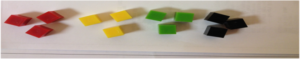Equal groups with quick pictures- With this strategy, students are still directly modeling what is happening in the problem, but they are now representing that model with a picture.Number Line- Students can make connections with their manipulatives and pictures to help them see how number lines can help them solve multiplication problems. The number of groups is represented by the number of jumps and the size of the groups is represented by the size of the jumps. The number at the end of the equal jumps represents the product.Repeated Addition- Students will begin making the connection that repeated addition will help them solve. When students use repeated addition they use the number that is in each group to add repeatedly. The number of groups will tell them how many times to add the number.

3 + 3 + 3 + 3

Skip Counting- As students understanding of multiplication progress, another strategy they will discover is skip counting. They will skip count the number that is in each equal group, and know when to stop based on the number of groups.

3, 6, 9, 12

Multiplication Sentence- All of the strategies discussed above will help students understand the meaning of a multiplication sentence. Once understanding of multiplication begins to build, students should be expected to write multiplication sentences that match their strategy.

4 x 3 = 12

Anchor chart that could be used as students discover the multiplication strategies:What can I do as the parent to support my child’s understanding of multiplication?

Encourage use of models. Make sure not to rush your child to solve without models. With repeated practice and exposure to multiplication, children will naturally become more efficient.

Ask questions that encourage your child to understand why they created the model they did or helps them think deeper about why they multiplied:
o How does your model relate to the problem?
o Equal groups models: What do your groups represent? What do the dots in each group represent?
o Number line model: What does each jump represent? How did you know the value of each jump? What does the number of jumps represent?

For more example division problems visit this website: https://www.ixl.com/math/grade-3/multiplication-word-problems

http://www.homeschoolmath.net/teaching/md/multiplication-number-line.phpWhat is fact fluency?

An important goal for third graders is to develop fluency with multiplication and division by the end of the school year. Fact fluency is the ability to think of numbers flexibly and respond accurately in about 3 seconds without resorting to non-efficient strategies (like counting on their fingers or drawing a picture).

Why should flash cards and timed tests be avoided?

Timed tests and flash cards encourage memorization of facts without understanding. Expecting students to memorize their facts without extensive practice with efficient strategies will lead to anxiety and frustration for students. Also, students who are being forced to memorize their facts with these tools will feel that there are many, many facts to learn without seeing the numerous relationship between the facts.What will lead to students developing fluency?

During the beginning of 3rd grade, the focus should just be on building understanding of multiplication and division. Students need to progress through the concrete, representational and abstract stages to develop a solid understanding of these two operations. The goal is to move students toward using more efficient strategies to solve, but they do not need to be fluent at this point. In the coming multiplication and division units, students will dive deeper into understanding and using efficient strategies that will begin to lead them toward fluency. These strategies will help students see the relationships between the basic facts.

For more information about fact fluency and why flash cards and timed tests should be avoided, check out these websites:
http://commoncore.tcoe.org/docs/default-source/Math-docs/cmc-building-multiplication-fact-fluencyEEE0AC828C38.pdf?sfvrsn=0

https://gfletchy.com/2015/08/17/not-your-moms-flashcards-conceptual-understanding-of-multiplication/

https://gfletchy.com/2014/12/01/from-memory-and-memorization-there-is-a-difference/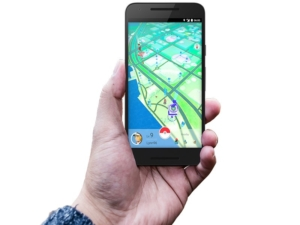We have all heard the term “accountable talk.” We get it, kids need to turn and talk to each other. They can’t sit and listen to us all day. But, how do we know that they are talking and learning? I know that we are supposed to walk around and listen and facilitate discussion, but what happens when we are talking to another group? How do you know that they are not talking about PokeMon Go? (Or anything else that is more important to them)

For many years, I thought that I was doing a great job of this. I was giving students the accountable talk stems, I was giving them time to turn and talk and then share out. So, why weren’t my students engaged in the learning that I was promised by all of the research?

The answer… quite simple. When I starting putting effective procedures in place for students to talk. DON’T STOP READINF YET!! I know… you think you already have the procedures in place. But, do you really?
Let’s look for example at a science investigation (since those are the most fun!!!)…

If students are working together and investigating, and you tell them to share with a partner what they just observed. Kids turn and tell their partner something and then they start talking about things that are off topic… Or, they both start talking at the same time and neither one is able to hear the other. In order for students to truly engage in meaningful conversation, there has to be “equity of voice.” And no it’s not just another buzz phrase… Stay with me on this.
Imagine if you had a procedure where on student shares what they observed and the other student than has to repeat what they heard and respond. They must discuss their reasoning for their response, and they hold each other accountable for talking and listening to one another. Like most things, it would take time and practice… but the conversations would be so much more student centered and based on what they were thinking.
Kagan has a great structure that could be used in any classroom.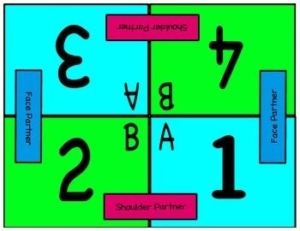When using these mats, you place them in the center of the student desks, and each student knows their number and letter. Before students turn and talk, you tell them that they are going to talk to either their face or shoulder partner, and that partner A or B is going to go first. After students have had a chance to share, the partner who was listening now has to repeat what they heard and then respond. Students never really know if they are going to talk to their shoulder partner or face partner, or who is going to talk first, so they must be ready to share at any point. They are also having to repeat what the other student said, and so they have to listen and think. You are now holding student more accountable for discussions.

After students have shared, they can either share again with the opposite partner (shoulder/face) or you can call on students to share out. This helps to change up the boring turn and just talk. They have a little bit more structure and will hopefully increase the quality of their conversations.

The first time you try this… maybe try it with something that you know they can talk about… Something simple, like what they did over the weekend. As you work through the barriers in your classroom you can add additional procedures as needed.
In case you don’t believe me… Here is some research!
“Accountable Talk® classrooms are filled with talk that seriously responds to and further develops what others in the group have said. It puts forth and demands knowledge that is accurate and relevant to the issue under discussion. This academically productive talk uses evidence appropriate to the discipline (e.g., proofs in mathematics, data from investigations in science, textual references in literature, documentary sources in history) and follows established norms of good reasoning. This discourse sharpens students’ thinking by reinforcing their ability to use and create knowledge.” (Michaels, O’Connor, Hall, Resnik, 2013)With our recent unscheduled days off thanks to Tropical Storm Hermine, students will no doubt be asking lots of weather related questions…
What is a hurricane and how do they form?
When will our next storm day be?
What is a Tropical Storm?
When will our next storm day be?
How did they know where the hurricane was going?
When will our next storm day be?

This is a great opportunity to take advantage of their curiosity through a Long-Term Investigation!!!!
Ideas for weather LTI’s include:

Storm Tracking – There are more storms developing daily. Your class can track them as they move using a tracking chart like this one. There are also lots of free resources out there online and locallyThere are 2 weather-related LTI’S ready to go on the Elementary Science Icon in the K-5 Curriculum Resources Folder under the icons:

Outside Our Window
When the Clouds Huddle
These LTI’s have standards connections for grades KG-5th, data collection charts, and graphing ideas to get your class LTI up and going and tap into student curiosity and interest!!!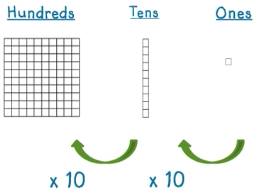Once upon a time long long ago… Just Kidding. A long time ago (like yesterday or the day before) a post came out about base ten and place value. If you didn’t get a chance to check it out just click on this link… http://ow.ly/BYwg3041trZ Its ok, I’ll wait.

Understanding this relationship between place value and multiplication and division we can solve problems more efficiently. Not only that, we will understand what is happening when we are solving these problems.

Lets take the number 77. The 7 in the tens represents 7 tens and the 7 in the ones represents 7 ones.In this model you can see that there are 7 groups of 1 and 7 groups of 10. This is going to help when we are applying multiplication and division because both represent groups. Examples below.

Lets take 2 x 3 or 2 groups of 3 as shown below.

So 2 groups of 3 or 2 x 3 gives us a product of 6. But if you look closely those each block actually represents 1,000.

So what would 2 groups of 3,00 equal? Well of course it equals 6,000. Using what we know about place value can help us not only multiply but also divide.

700 divided by 70. I know that there is a 7 in the hundreds place in the number 700 and a 7 in the tens place in the number 70. If I know that each place value to the left is ten time greater than the place value to the right, as shown below.That mean that 700 is ten times greater than 70 or it would take 10 groups of 70 to equal 700. This could be written as 700 divided by 70 equals 10.

This structure of ten times greater when you move up each place value and ten times less when you move down each place value can really help when multiplying and dividing larger numbers.

For more information on Understanding place value: multiply by a power of 10 check out Learnzillion.com https://learnzillion.com/lesson_plans/6646

For more information on understanding relationships between digits and their place value check out Learnzillion.com https://learnzillion.com/lesson_plans/7426Top 5 Things to know about using arrays to model multiplication & division:
1. An array is an arrangement of things organized into equal rows and equal columns, which creates a rectangular shape. The rows represent the number of groups and the columns represent the number in each group.Tools that are good for modeling arrays include: counters, square tiles, graph paper, and quick pictures. Using arrays to model division or multiplication problems, we want to move from concrete manipulatives, to quick pictures, to abstract thinking using expressions or equations to describe the arrays.

This is a concrete model of an array. This could be the model of a division problem involving 15 ÷ 5 or 15 ÷ 3. This could also be the model for the multiplication problem 5 x 3.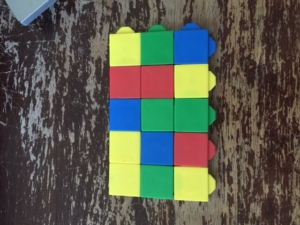A graph paper array.Quick picture of an array with the related equations.2. Arrays can be used to model both multiplication and division problems. They help relate to the two operations.

Here is an example of using a multiplication array to relate to division. Once the total is determined, students can see they can divide by the number of groups or rows, 2. Or they can divide by the number of columns or amount in each row, 3.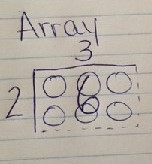3. It’s beneficial to use word problems that elicit organizing an object into equal rows or columns to encourage students to model an array.

Example Multiplication Problems:
• “Riley was planting in his tomato garden. He planted 3 rows of tomato plants. There were 5 plants in each row. How many tomato plants did Riley plant in his garden?”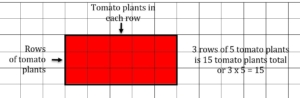• “Riley was planning in his tomato garden. He planted 3 tomato plants in each row. He planted 5 equal rows of tomatoes. How many tomato plants did Riley plant in his garden?”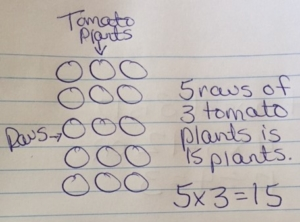Example Division Problems:
• “Rachel has 15 dolls. She has 5 shelves to place her dolls on. She wants an equal number of dolls on each shelve. How many dolls does Rachel place on each shelf?”• “Rachel has 15 dolls. She places 5 dolls on each row. How many rows does Rachel need to place all of her dolls on a shelf?”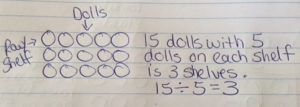4. Arrays help teach the commutative property of multiplication, which leads students to more efficient strategies for multiplying fluently.

This model shows 3 rows of 5, or 3 x 5.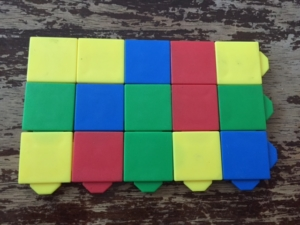This model shows 5 rows of 3, or 5 x 3Based on these two models, students can see the total or product stays the same because you are not adding or taking away any square tiles. The only thing that has changed is the way the rows and columns are organized, or the number of groups and how many in each group.

5. Array understanding in 3rd grade is essential for students developing understanding of area later in 3rd grade, and further in 4th grade. It also prepares students for modeling larger multiplication problems using partial products in 4th grade.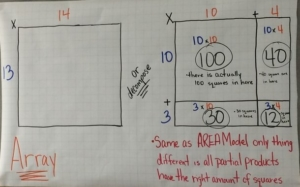A fun way to explore modeling arrays with multiplication and division problems is to give students bingo stampers and have them stamp the arrays on construction paper. You could pose the task, “There were 24 students that needed to be organized into equal rows for the assembly. How might their teacher have organized them into equal rows?” Then encourage students to record the division and multiplication equations for each array model.A great game to encourage students to practice modeling arrays is the “Array game.” Give partners a half sheet of graph paper, two different colors of crayons and a 0-9 number cube. Have the students take turns with their partner, rolling the number cube twice to determine the number of rows, and number of columns for their array. Then they will create their array, recording the equation that matches it and shading it their color. Play continues until a person can no longer create their array. Then the winner is whomever has the most square units shaded in their color crayon. (This can also be modified for division by creating division problem cards that students can pull, then create the array to match the given problem and solve for the quotient).

For more information on using arrays to model with multiplication and division problems or lessons, visit the websites:
http://investigations.terc.edu/library/curric-math/qa-1ed/teaching_mult_div.cfm
https://learnzillion.com/lesson_plans/5215-solve-division-problems-using-arrays
https://nrich.maths.org/8773
https://www.eduplace.com/math/mw/background/3/08/te_3_08_overview.html

For a lesson incorporating the use of arrays on CPalms, visit this website.What Do You Mean Base 10?

Well our entire place value system is based on 10. In the visual below it shows how it would take 10 ones to make 1 ten. It would then take 10 tens to make 1 hundred, and 10 hundreds to make 1 thousand. This pattern continues indefinitely.Now that we have seen the model that represents this relationship between place values it’s time to put it to work! Have you ever heard anyone say “that’s going to cost 11 hundred dollars?” (it could be 14 hundred or 18 hundred… you know what I mean.) They are referring to the amount of hundreds. In the example of 11 hundred, it could also be read as 1 thousand 1 hundred.

So which way is right?

The answer is they are both right. 11 hundred is the same as 1 thousand 1 hundred. We want students to be able to use these interchangeable.

4th graders will be representing numbers through the millions as shown with this place value chart below.They will be using many of the strategies they have learned in previous grade levels about the relationship between place values. In the example below we have created a number.If were to ask for the value of the 4 in 4,532 a common mistake would be the answer 4. Even though 4 is the digit we are looking for the value, and that is determined by the digit’s place. Below you will see an example.

The 4 is actually worth 4,000 because the digit 4 is in the thousands place.

For more information on this relationship between place values check out this lesson on Learnzillion.com https://learnzillion.com/lesson_plans/8993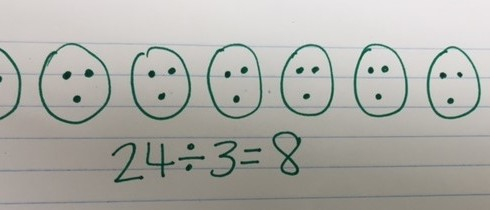What is division?

Division is when you know the total amount, and either the number of groups or the size of the equal groups are unknown. Students are expected to understand this process looking at the actions in a story problem and direct modeling what happens to find the quotient. This is presented in various ways to ensure students understand what division is and how it’s related to multiplication.

How should division be introduced?
• Direct modeling with manipulatives – We want students to understand division, so it’s important to have them directly model the actions in a problem using first concrete manipulatives such as counters and then move to drawing quick pictures to directly model the actions in a problem. As students are directly modeling, we want to pose questions to get them to think deeper about what’s happening and how they got their answer. Questions to ask include:
o What do your groups represent?
o What does the dots/cubes in each group represent?
o How does your model relate to the story problem?

Direct Modeling with Counters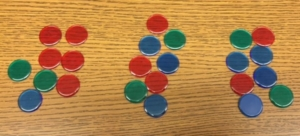Direct Modeling with Base-ten blocks• In Context – This occurs when you use story problem scenarios that represent division. A story problem could include, “Mark has 24 apples. He gave an equal amount to 3 of his friends. How many apples did each friend receive?”
• Out of Context – This occurs when you present students with an expression to solve, such as 24 ÷3. They can then solve the problem by putting it into context of a story problem to understand the actions.
• Relate to Multiplication – As students are exploring division, they will naturally start seeing similarities to multiplication and division. It’s important to encourage and facilitate these discussions. Some observations may include:
o There are equal groups in multiplication and division
o With division I know the total, with multiplication I don’t
o The model for 24 ÷ 3 looks the same as my model for 3 x 8

How does measurement and partitive division compare?

Measurement and Partitive Division are two types of division that occur based on the type of problem presented. It’s important for children to decontextualize, or break down the problem using the model that directly models the actions in the problem and also represent it with an expression or equation. We want to make sure we expose students to each type of problem, and have them verbalize what they know and what they are trying to find out.

Measurement Division – This type of division occurs when you know the total and how many in each group, but the number of groups is unknown.

Example problem, “Mark has 24 apples. He gave an equal amount to 3 of his friends. How many apples did each friend receive?”

First you would count out 24 “apples” or counters.Next you would begin separating the “apples” or counters into 3 equal groups.You would continue to separate the counters into the 3 equal groups until they are either all used or you can no longer create equal groups, then you would have a remainder (which is not addressed in 3rd grade math standards).Partitive Division – This type of division occurs when you know the total and how many groups, but the number in each group is unknown.

Example problem, “Mark has 24 apples. He gave 3 apples to each of his friends. How many friends received 3 apples?”

First you would count out 24 “apples” or counters.Next you would begin separating the “apples” or counters into equal groups of 3 apples.You would continue to separate the counters into equal groups of 3, until they are either all used or you can no longer create equal groups, then you would have a remainder (which is not addressed in 3rd grade math standards).You want to use the same numbers for partitive and measurement division problems, encouraging students to compare and contrast the two division types. This helps to move students from thinking “I’ll get the same answer anyway,” to “which strategy really directly models the actions in the problem.” This will also help students deepen their understanding of what division is.

What models and strategies can students use to demonstrate an understanding of division?
• Equal groups model with manipulatives – Using Counters or Base ten blocks

I have 24 counters total to separate into equal groups.I was able to separate the 24 counters into 3 equal groups of 8, so the quotient is 8.Base ten block models can also be used, which can be a more efficient strategy for modeling division.

• Equal groups quick picture model – It’s important for students to draw quick pictures, not detailed models

First you begin with create a base ten model of 24, then draw the three groups you will evenly separate the base ten blocks into. You can place one unit into each group.Next you realize you have to break-apart one of the tens into ones to continue to separate the 24 into equal groups.Finally you separate all of the 24 into equal groups. The answer is then 8 which is the quotient, the amount that is in each equal group.• Number lines – Where you begin with the total, the jumps represent the groups, and the numbers within each jump represent how many in each group.

We began with 24 and then made equal jumps of 3. There were 8 jumps, or equal groups. So 8 is the quotient.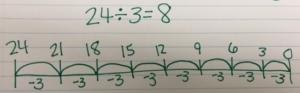• Arrays – An arrangement of things in rows and columns into a rectangular shape. This can be used with division and multiplication. (There will be another blog that goes into greater detail with this model).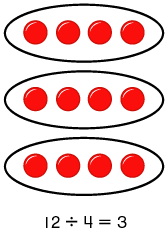What can I do as the parent to support my child’s understanding of division?
• Encourage use of models. Make sure not to rush your children to solving without models, we want to ensure understanding. With repeated practice and exposure to division, children will naturally become more efficient.
• Ask questions that encourage your child to understand why they created the model they did or helps them think deeper about why they even divided:
o How does your model relate to the problem?
o Equal groups models: What do your groups represent? What do the dots in each group represent?
o Number line model: What does each jump represent? How did you know to begin with the total? What does each number in each jump represent? Why are the numbers decreasing rather than increasing?
o Array models: What does each row/column represent?

For more example division problems visit this website. For more information on understanding division with models visit this websiteWe are all aware of the CRA model of providing students experiences with mathematics content. In terms of exponents, students very rarely experience the “C” (concrete) or the “R” (representational) and usually begin with the “A” (abstract). So how can we provide “C” and “R” experience?

Last week we shared a PowerPoint from CPalms with images for students to look at powers of ten. This blog post shares pictures of students using manipulatives to model the exponential value.Students should be familiar with base ten blocks. Which of these blocks could be used to represent 102? Let’s start by thinking which represents 10? (The ten rod/long) If the exponent 2 or “squared” means to multiply 10 x 10, how can we represent that? That’s right, a hundred flat. When you look down upon a flat, you even see the shape of a square!

Let’s move to 103. What multiplication problem are we talking about here? (10 x 10 x 10) How can we show that with blocks? We know a flat represents 10 x 10, so we need 10 flats. That’s one of the large cubes! We can begin to see a pattern: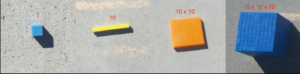What exponents could we use to label our base ten blocks? What exponent works for the ones cube?
How can we represent 104? Each time we move to the next exponent of ten, we are basically creating another group of 10 of that model. So what would ten groups of large cubes look like? How about ten groups of that object for 105? This is a great challenge for students to represent both physically and through drawing.
Below are images one of our math coaches have captured from student work in this area: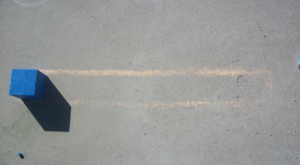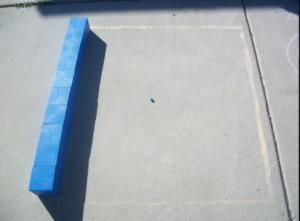Here are some additional questions you can use with your students: What patterns do you see? What does the picture from the beginning of the blog post represent? How many ones cubes fit in each of these models? So what is the value of each of these new “super” base-ten blocks?

Making and Recording Observations

As scientists one of the process skills that we must use is making observations. Why? We must be able to communicate what we have discovered using our senses and tools. “These observations often serve as evidence in the scientific arguments, explanations, and theories of the field. Like scientists, students engage in these practices to learn about the disciplinary core ideas and crosscutting concepts as called for by the Next Generation Science Standards.” (Arias and Davis, 2016)
So… what does it look like when students are making observations? Observations need to have certain characteristics:
• They need to be clear- another scientist may need to use their information later
• They must be complete- students should record all necessary details relevant to the investigation- think senses… Which of the five senses, or all can they observe and record.
• They should be accurate- duh! The observations should be what they actually heard, smelled, or saw… not what they inferred or thought they saw, heard, and smelled.
• Try to be objective- Not that their car rolled far, but how far? Was in 10cm or maybe even 100 cm?
• Observations should be well-labeled and using scientific vocabulary. Remembering again that anybody should be able to interpret their observations.

I know what you’re thinking: “that sounds great but not all students are successful at making observations.” Let me tell you what research says about that: yes, there are struggles. Some of these common struggles are that students think they will remember their observations so why do they have to write them down: Believe me, they will forget! (Don’t let them convince you that they won’t) Students also record inferences rather than observations. You may need to remind students that observations are only things that can be seen, heard, smelled, and touched. Inferences are what they believe is happening based on their observations. One of the other struggles is that students are not recording observations that are clear, complete, and objective. As teachers we need to ensure that we are holding students accountable and have a working plan in place for scoring observations.

This is when rubrics come into the equation. One way to have student buy in with rubrics is to have them help you create the criteria for the rubric.Have students decide what they think should be the criteria in these boxes. This can change from time to time and may need to be adjusted depending on the goal you have in mind.

Lastly, when you are looking at student’s observations they should be given feedback. If all they do is put their observations in their notebook and nobody ever looks at it, well…. They are pretty clever things and will not be ensuring that they have all the components and criteria that THEY agreed they should have.

Like always, you have to think about the needs of your students and how you are going to meet their needs. Do what is best for your students!!

HAPPY OBSERVING!!

References
Arias, Anna Maria., Davis, Elizabeth A. (2016) Making and Recording Observations. Science & Children (53). Pages 54-60.Do you struggle teaching powers of ten? Do I only teach it as a procedure or memorization of rules?Check out the link below from CPALMS. The lesson shows an engaging way to support students in making sense of powers of ten without memorizing rules. The lesson includes 5 different attachments including a PowerPoint that you could easily use in your classrooms to engage students in understanding powers of ten along with notes for each slide. Have fun and enjoy watching your students make sense of mathematics!!!

http://www.cpalms.org/Public/PreviewResourceLesson/Preview/47989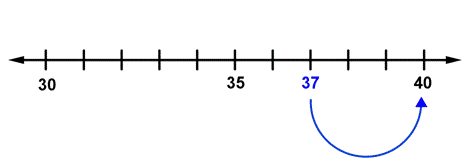Five or more raise the score, four or less give it a rest! This saying and others similar to it are often used to help students remember how to round numbers. When these students are asked why they rounded 48 to 50, they explain that the 8 in the number 48 is five or more, so they raised the score. They are not able to explain that because 48 is only 2 away from 50, but is 8 away from 40, 48 should be rounded to 50. Open number lines can help students build conceptual understanding of how to round numbers.How to get students to use open number lines when rounding:
To build understanding of using open number lines to round, engage students with a human number line. Challenge students to round the number 76 to the nearest 10. Write the number 76 on an index card. Use a string (that 2 students hold) and have a student place the index card on the string with a clothes pin. Pose the question “What are the two closest tens to 76?” Students place the numbers 70 and 80 on either side of 76. Finding the mid-point between 70 and 80 will also help students know exactly where 76 should be on the number line. Ask students “Which ten is 76 closest to?” They will be able to see that 76 is closer to 80. They should also be able to explain that 76 is 4 away from 80.

After practice with a human number line, some students will be able to sketch an open number when rounding. Continue to expose students to this strategy when sequencing students while sharing their work.Here are the Top 5 things to consider when teaching 3rd grade students about adding and subtracting multi-digit numbers with place value understanding:

1. Student’s prior knowledge from 2nd grade and how it relates to 3rd grade standards

Students in 2nd grade were expected to…
• Fluently add and subtraction within 100 using strategies based on place value
• Add up to four two-digit numbers using strategies based on place value
• Mentally add/subtract 10 or 100 to a given number between 100-900
• Explain why addition and subtraction works

Students in 3rd grade are expected to fluently add and subtraction within 1,000 using strategies and algorithms based on place value.

2. Use of models to build conceptual understanding

Though students have learned addition and subtraction with a variety of strategies and models before they get to you in 3rd grade, it’s important to still encourage the use of models to deepen the conceptual understanding of what addition and subtraction is with larger numbers. This will encourage the use of place value language, which is necessary in understanding the process of addition and subtraction of multi-digit numbers within 1,000. Models may include use of base ten blocks, quick pictures or number lines. As you connect and compare these different strategies by posing questions to students, the understanding of addition or subtraction will deepen.

Base ten blocks model example:Base ten Quick Picture Example: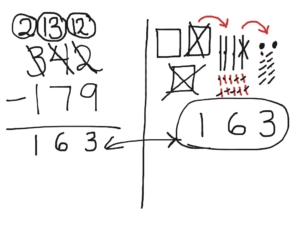Adding with a Number Line Example: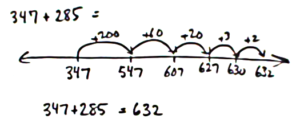3. Importance of explaining the addition or subtraction process with place value language

As students create models, solve with algorithms or invented strategies, it’s important to encourage the use of place value vocabulary or language to describe the steps taken to solve. This ensures that they will have a deep conceptual understanding of addition or subtraction and not just the procedure of solving a problem.

For example a student may solve the problem 216 + 372 by describing, “I added the six ones and 2 ones, so now I have 8 ones. Then I added the 7 tens and 1 ten to get a total of 8 tens. Last I added the 2 hundreds and 3 hundreds to get a total of 5 hundreds. With the 5 hundreds, 8 tens and 8 ones I have a total of 588.” This is much different than having a students say, “First I added the 2 and 6 to get 8. Then I added the 7 and 1 to get 8. Then I added the 3 and 2 to get 5.” The second explanation may create some common misconceptions about what each digit really represents and how it creates the value of the solution.

4. Presenting problems in context with story problems to encourage students to “choose an operation”

Understanding the structure of addition and subtraction is one piece to the puzzle. The other big piece is to have students determine whether they are adding or subtracting with a given story problem or scenario. In order for this to happen you pose problems, explore and share thinking about what actions in the story problem told you to add or subtract. (AVOID KEY WORDS AND FOCUS ON KEY ACTIONS). This can also be done by having students complete a problem sort by:
• Give multiple story problems on index cards/slips of paper/etc.
• Have students justify whether they would use addition or subtraction to solve and why (may include directly modeling the problem to prove their thinking)
• Place each problem into the addition or subtraction pile to show their choice

There are even some problems where they could use either addition or subtraction, depending on how you look at the problem. The most important thing is that students explain why they chose the operation they did and how they will use that operation to solve the problem correctly. For example: Tony had 715 apples total. He started with 342. Then he and his friends picked some more together. How many apples did Tony and his friends pick together?” It’s important to have a balance of the understanding of how addition/subtraction works and how to use the operations in real-world problem solving scenarios.

Students also need to break down the problem to make sense of it with numbers. They also need to determine how the numbers they are using to solve the problem relate back to the original story problem. This can be encouraged by posing questions like, “How does this number relate to the problem? How does your expression match the actions in the problem?” (This is also known as contextualizing and decontextualizing).

5. Expose 3rd grade students to a variety of problem types

It’s important that students see various problem types to prepare them for the real world. Not all problems will be presented the same way and they need to be prepared to apply the problem solving strategies they learned with any problem structure they encounter.

Problem types are listed in this Common Core Chart below:To reinforce addition of numbers to 1,000 visit https://goo.gl/Uanlr2. To reinforce subtraction of numbers to 1,000 visit https://goo.gl/fUwWhg.

Another great website to encourage the use of base ten models with the “base blocks” virtual manipulatives listed to solve addition and subtraction problems is: http://nlvm.usu.edu/en/nav/grade_g_2.html.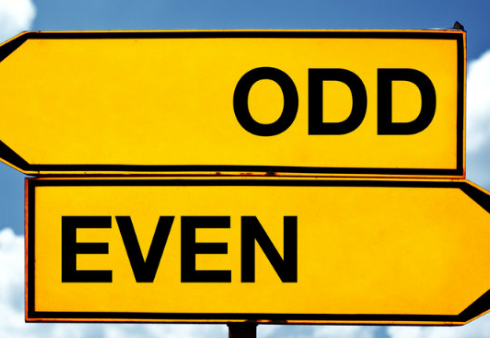If you add two odd numbers will your answer be an even or odd number? What about two even numbers? Will those results always be true? The idea of evens and odds may seem simple but it is a powerful foundational concept. They are found everywhere in our daily lives. Shoes and socks are sold in pairs. Football and basketball teams play with an odd number of players from each team. But what is the big deal? Why is it important for our kids to know this concept? Well, children will use the idea of equal groups as a foundation for multiplication in third grade.

There are multiple ways for children to gain a strong understanding of evens and odds. Separating groups of objects into groups of 2 or separating into 2 equal groups with none left over are two important ways kids learn even. If there is one item left over the amount is odd. It builds lasting understanding when child explore with objects and begin to find patterns. Children will then make the connection between the concrete objects to drawn picture representations and later relate this concept to the doubles addition facts (i.e. 8 + 8 = 16 or 3 + 3 = 6).

Here are a few ways that pairs of objects can be organized or placed in two equal groups.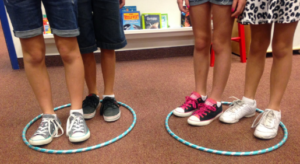To play “Find Odd or Even game” try out this link. Encourage the use of objects or drawings to represent the amounts.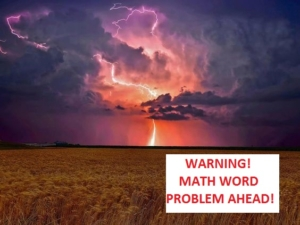Students of all ages have difficulty applying comprehension skills when reading and interpreting math word problems. Those of us who attended school before the year 2000 know that most of our instruction was computation with two word problems to solve at the end. Math instruction looks different today as it should. Years of computation without application to real world contexts have created masses of adults who readily admit they hate math, aren’t very good at it, or worse, are afraid of it.

We now purposely immerse students in understanding the context of a word problem to apply those computation skills, but with this can come frustration in not know what the problem is asking. Too many times students just grab the numbers in a problem and operate on them. To test this out, give this word problem to a fifth grader and tell them it is in another language. Ask the student to solve it, and watch what happens.

Siejg ejk wla 60 akylhl ssy 2 anklh, wyp whnzy pog aliy ume?

Did the student say the answer was 120? 62? 58? 30? No matter the age of the student, it seems their innate behavior is to add, subtract, multiply or divide without caring to read the situation. On a poorly written assessment that is solely focused on one operation, this strategy may work well, but on a well written assessment, this behavior will lead the student to undesirable results.
Fortunately there are graphic organizers that we can use to teach students how to comprehend a math word problem. One of those is called the KWPPR (Sue O’Connell, Mastering the Basic Facts).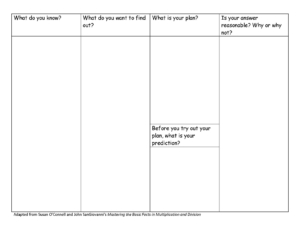The first column of the organizer poses the question: “What do you KNOW?” After reading the word problem, students identify what they know in the problem. In other words, they identify what the facts are that are important to solving the problem. Sometimes they are not sure what is important or not so they list all of the facts they find from the problem.

The second column poses the question: “What do you WANT to find out?” Students then identify what the question is. What is the problem asking? They write that in the chart.

The third column has two parts: “What is your PLAN?” and “What is your PREDICTION?” First, for the plan, students write what tools they will use or what operation or strategy they will use to solve the problem. For example, they might use partial products to solve a multiplication problem. Second, what is my PREDICTION? Estimating answers builds reasonableness so this is where students will predict what they think their answer may be close to or at least if the number will have a greater value or lesser value.

The fourth and last column poses the question: “Is your answer REASONABLE?” Sometimes teachers change that last column to “Does your answer make SENSE?” This is where students show their thinking and explain it. Fifth graders should be able to explain their thinking in a way that convinces others that they are correct and that they understood the problem.

Here is an example of how the KWPPR can be used to solve the following problem.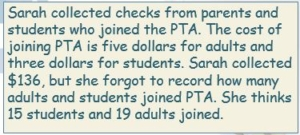Try it out with students!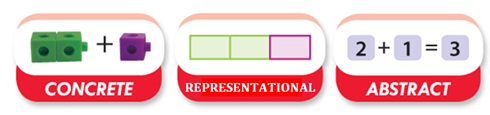What is the Concrete-Representational-Abstract (CRA) instructional approach?
CRA is a gradual systematic approach to teaching mathematics. Each stage builds on to the previous stage and therefore must be taught in sequence. This three step approach has been found to be highly effective in teaching math concepts.Concrete Stage:
The first step is called the concrete stage. It is known as the “doing” stage and involves physically manipulating objects to solve a math problem. It is critical that that students use manipulatives that represent the concept being studied. Students must be fluent in manipulation of the concrete materials and must be able to explain the connection to the concept or procedure.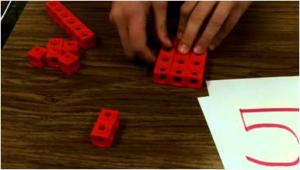Representational Stage:
At the representational level of understanding, also known as the “seeing stage”, students learn to problem-solve by drawing pictures, models, charts, etc. The visuals represent the concrete objects students manipulated when problem-solving at the concrete level. Not all students will progress to the representational stage at the same rate. Some students may need more time at the concrete level.Abstract Stage:
The final step in this approach is called the abstract stage. It is known as the “symbolic” stage and involves using only numbers and symbols to solve a math problem. Students who struggle to solve problems at the abstract level often need to spend more time building understanding at either the representational or concrete level.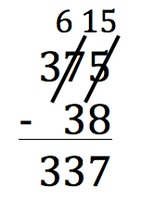How to move students from concrete to representational:
Provide scaffolding by asking students to draw pictures to show what they have done with the manipulatives. This can be a simultaneous process until students are comfortable making representations without the use of the manipulatives.

How to move students from representational to abstract:
Ask students to write numerical expressions to match their drawings (i.e. number sentences). Students may continue to draw pictures until they are comfortable in the abstract level.

How does CRA relate to Unit 1 in 3rd grade?
In Unit 1 (Addition and Subtraction within 1,000) the standard states that students will be able to fluently add and subtract within 1,000 using strategies and algorithms based on place value, properties of operations, and/or the relationship between addition and subtraction (MAFS.3.NBT.1.2). We know that students used the algorithm to add and subtract in 2nd grade, so we often think they should automatically be able to use the algorithm in this unit as well. In 3rd grade, it is still important that we allow students to progress through the CRA stages so they will have conceptual understanding of the standard.

For more information about CRA instruction, visit these websites: https://makingeducationfun.wordpress.com/2012/04/29/concrete-representational-abstract-cra/ and http://www.coedu.usf.edu/main/departments/sped/mathvids/strategies/cra.html . Also, there is a Mini PDM located on the math icon in IDEAS that is focused on CRA.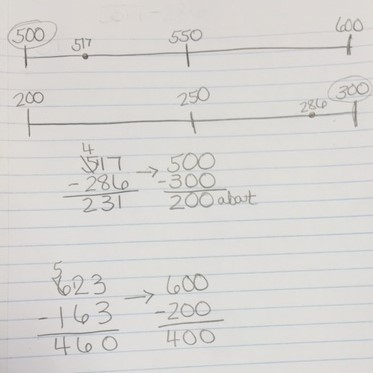What is estimation?
Estimating is an important skill. Estimation is the process of getting an approximate amount, sum or difference. We want students to be able to use mental math to more quickly to arrive at a reasonable answer for their sum or difference. In 3rd grade, students are adding and subtraction fluently within 1,000.

What are different ways to estimate?
• Front-end estimation is a reasonable strategy to estimate sums and differences when all or most of the numbers have the same number of digits. To use this strategy, add or subtract the front digits of the number (or the digits in the greatest place), then make adjustments based on the numbers in the next greatest place.

Example: 345 + 416 + 298. Add the 3, 4, and 2 hundreds (numbers in the greatest place) to get an estimate of 900. Adjust by adding the numbers in the next greatest place which are 4, 1, and 9 tens to get 140. 900 + 140 gives you a front-end estimation of 1,040.

• Compatible numbers is a useful strategy when adding more than 2 addends, or typically a long list of numbers. With this strategy, you look for 2 or 3 numbers that can be combined to create 10 or 100 to make it easier to determine an estimate.

Example: When adding 18 + 39 + 71 + 27 + 78 + 62, I can estimate the sum as 300 by combining numbers that estimate to 100: 18 and 78; 39 and 62; 71 and 27.

• Rounding is used to determine the nearest 10 or 100 to the whole number to estimate a sum or difference, many times to give you closer approximation.

Example: 328 + 74. Rounding to the nearest ten I estimate 330 + 70 = 400.

Why is it important to estimate sums and differences?
Estimation is an important skill to utilize without being prompted. It helps students determine reasonableness of their solution. This should occur before students actually solve for the sum or difference, and then compare the actual solution to the estimate students need to solve again for accuracy. Without estimation skills, students aren’t able to determine if their answer is within a reasonable range.

Estimation is also an important life skill. When you are shopping and you want to stay within a budget, you are more likely to estimate the cost of all the items you want to purchase than to pull out a calculator to determine the exact amount, including taxes while standing in the store. We want students to see important connections to math and everyday life. This happens in the classroom as we connect real-world using problem solving scenarios.

How do I help my students/child explore and develop an understanding of estimating sums and differences?
• Practice estimating whole numbers by using a number line.
The use of a number line is essential when exploring estimating sums and differences. You want to begin with students determining the range for one of the numbers involved. Then determine the middle number. From there, place the location of the number on the number line. Determine which of the range numbers the whole number is closest to, that becomes the estimated number.

For example: Below is an example of estimating 37. The student determined the range of 30-40 for the number line. The middle number is 35. The location for 37 was placed and it was closer to 40. That means 37 is estimated to be 40.This can also be done by pre-making different ranges of number lines on index cards. Place a number under the elmo or on the board and have students “put their heads together” to determine and justify which range would be used to estimate the given number, what the middle number would be, what each individual number is estimated, based on the numbers’ location on the number line, and the estimated sum or difference.

Below is an example of possible number lines to estimate the given addition problem.The range of 300-400 was selected for the number 367. The range of 500-600 was selected for the number 516. From there the student determine the middle numbers and the location of each of the numbers on that number line to determine 367 is estimated to 400, and 516 is estimated to 500.

From there, the students adds the two numbers to estimate the sum at 900. When the student actually finds the sum of the original addition problem, they can see that their estimate is reasonable.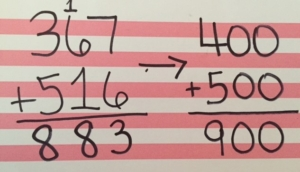• Apply the estimation to determining sums and differences as a strategy for determining reasonableness of an answer.
After students understand the concept of estimating, have them apply that understanding to addition and subtraction problems. Students need to understand that it is a necessary step to determining if their end solution is reasonable, and should continue to use this skill even when it’s not prompted to do so.Eventually students will get to the point where this can happen mentally as shown in the example below.• Solve problems that require estimation as the answer.
Students need to understand that sometimes the estimated sum or difference becomes the answer. They don’t need to solve for the actual solution with these problems.

An example problem may include, “Tonya has \$812 in her checking account. She wants to go shopping to purchase a purse for \$316 and a pair of jeans for \$78. Does she have enough money? If so, about how much will she have left in her checking account?”

Read the article located at http://goo.gl/x3JviT for more information on why it’s important to focus on the skill of estimation with students.

Explore the virtual manipulatives Place Value Number Line. This manipulative requires students to drag a value to its appropriate place on a number line. The endpoints of the number line can be adjusted using the place tab in the bottom left corner of the screen. Students can use this tool to identify “nice” or compatible numbers.Estimation is often overlooked in lessons involving multiplication or division. Even if students are told to estimate first, they often calculate to find the precise answer then round it to make an estimate.That should prompt teachers to consider the purpose of estimation. Are we doing students a disservice if we bypass estimating before computing? According to industry leaders, estimation is the single most critical skill to apply in planning for land development, architectural and engineering design, and project cost development. Estimating the time it will take to complete a project is a critical skill. If we survey shoppers at the mall, how many will say they pause shopping to calculate the precise answer or estimate costs?

For elementary age students, estimation is a tool to monitor reasonableness of answers.

In the Common Core State Standards for Mathematical Practice, using appropriate tools strategically emphasizes students using estimation prior to computing. “Students detect possible errors by strategically using estimation and other mathematical knowledge.” Click here to learn more! Standards for Mathematical Practice

As you watch the following video, consider the following:
• How is estimation used as a strategy for solving division problems?
• How beneficial is it for students to use estimation as a strategy rather than only finding an estimate as answer?
• How can estimation and adjusting the quotient help when dividing with 2-digit divisors?

Video: Divide 4-digit dividends by 2-digit divisors by estimating and adjusting the quotient